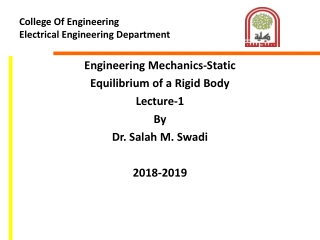DownloadDownload PresentationCollege Of Engineering Electrical Engineering Department

# College Of Engineering Electrical Engineering Department

Télécharger la présentation## College Of Engineering Electrical Engineering Department

- - - - - - - - - - - - - - - - - - - - - - - - - - - E N D - - - - - - - - - - - - - - - - - - - - - - - - - - -
##### Presentation Transcript

1. College Of Engineering Electrical Engineering Department Engineering Mechanics-Static Equilibrium of a Rigid Body Lecture-1 By Dr. Salah M. Swadi 2018-2019

2. Chapter Objectives • Develop the equations of equilibrium for a rigid body • Concept of the free-body diagram for a rigid body • Solve rigid-body equilibrium problems using the equations of equilibrium

3. Chapter Outline • Conditions for Rigid Equilibrium • Free-Body Diagrams • Equations of Equilibrium • Two and Three-Force Members • Free Body Diagrams • Equations of Equilibrium • Constraints and Statical Determinacy

4. 5.1 Conditions for Rigid-Body Equilibrium • The equilibrium of a body is expressed as • Consider summing moments about some other point, such as point A, we require

5. 5.2 Free Body Diagrams Support Reactions • If a support prevents the translation of a body in a given direction, then a force is developed on the body in that direction. • If rotation is prevented, a couple moment is exerted on the body.

6. 5.2 Free Body Diagrams

7. 5.2 Free Body Diagrams

8. 5.2 Free Body Diagrams Internal Forces • External and internal forces can act on a rigid body • For FBD, internal forces act between particles which are contained within the boundary of the FBD, are not represented • Particles outside this boundary exert external forces on the system

9. 5.2 Free Body Diagrams Weight and Center of Gravity • Each particle has a specified weight • System can be represented by a single resultant force, known as weight W of the body • Location of the force application is known as the center of gravity

10. 5.2 Free Body Diagrams Procedure for Drawing a FBD 1. Draw Outlined Shape • Imagine body to be isolated or cut free from its constraints • Draw outline shape 2. Show All Forces and Couple Moments • Identify all external forces and couple moments that act on the body

11. 5.2 Free Body Diagrams 3. Identify Each Loading and Give Dimensions • Indicate dimensions for calculation of forces • Known forces and couple moments should be properly labeled with their magnitudes and directions

12. Example 5.1 Draw the free-body diagram of the uniform beam. The beam has a mass of 100kg.

13. Solution Free-Body Diagram

14. Solution Free-Body Diagram • Support at A is a fixed wall • Three forces acting on the beam at A denoted as Ax, Ay, Az, drawn in an arbitrary direction • Unknown magnitudes of these vectors • Assume sense of these vectors • For uniform beam, Weight, W = 100(9.81) = 981N acting through beam’s center of gravity, 3m from A

15. 5.3 Equations of Equilibrium • For equilibrium of a rigid body in 2D, ∑Fx = 0; ∑Fy = 0; ∑MO = 0 • ∑Fx and ∑Fy represent sums of x and y components of all the forces • ∑MO represents the sum of the couple moments and moments of the force components

16. 5.3 Equations of Equilibrium Alternative Sets of Equilibrium Equations • For coplanar equilibrium problems, ∑Fx = 0; ∑Fy = 0; ∑MO = 0 • 2 alternative sets of 3 independent equilibrium equations, ∑Fa = 0; ∑MA = 0; ∑MB = 0

17. 5.3 Equations of Equilibrium Procedure for Analysis Free-Body Diagram • Force or couple moment having an unknown magnitude but known line of action can be assumed • Indicate the dimensions of the body necessary for computing the moments of forces

18. 5.3 Equations of Equilibrium Procedure for Analysis Equations of Equilibrium • Apply ∑MO = 0 about a point O • Unknowns moments of are zero about O and a direct solution the third unknown can be obtained • Orient the x and y axes along the lines that will provide the simplest resolution of the forces into their x and y components • Negative result scalar is opposite to that was assumed on the FBD

19. Example 5.5 Determine the horizontal and vertical components of reaction for the beam loaded. Neglect the weight of the beam in the calculations.

20. Solution Free Body Diagrams • 600N represented by x and y components • 200N force acts on the beam at B

21. Solution Equations of Equilibrium